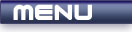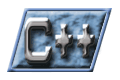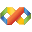Home Latest News Archive Tutorials C++ 2 3 4 Win32 Java OpenGL GLUT OpenGL ES 2 3 4 DirectX VB.NET HTML Photoshop 3dsmax Forums Projects Modelling Links About Us Contact Search Recent Tutorials C / C++ Win32 OpenGL OpenGL ES GLUT## References

Tutorials > C++ > References

### What are references?

A reference is basically an alias for a variable. It allows more than one variable to reference the same piece of data in memory or on the stack.

### How do references differ from pointers?

Pointers point to a part of memory. A pointer is a memory address. A reference is an actual variable and can be used without dereferencing the variable.

### How are references declared

References take the form :

data type &variable name;

Contents of main.cpp :

```#include <iostream>
#include <stdlib.h>

using namespace std;

int main()
{
```

In our example below, a reference y is created and is made to reference x. A reference can only be set to reference a variable when it is declared. In other words, y can now not be used to reference any other variable but x.

```	int x = 5;
int &y = x;
int z = 20;
```

We now increase x by 10. When printing out the values of x and y, we find that they both equal 15. This is because they both reference the same data in memory. If you change the value of one, you change the value of the other.

```	x += 10;

cout << "x : " << x << endl;
cout << "y : " << y << endl;
```

Remember me saying that a reference can never reference another variable? In the example below, z is assigned to y. This does not make y reference z. It only assigns the value of z to y. Because y references x, x now also has the value of z.

```	y = z;

cout << "x : " << x << endl;
cout << "y : " << y << endl;
```

To try and make things more clear, you can output the memory address of each variable. You will notice that x and y reference the same memory address where z has a different memory address. This strongly shows how references work.

```	cout << "x addr : " << &x << endl;
cout << "y addr : " << &y << endl;
cout << "z addr : " << &z << endl;

system("pause");

return 0;
}```

You should now have a basic understanding of what references are and how they can be used. You may also be wondering why you would ever need references. An example is shown in the next tutorial.

Please let me know of any comments you may have : Contact Me

 Source Files :< Tutorial 21 - Function Parameters Tutorial 23 - Passing Parameters >